# The Omnitruncated 24-cell

The omnitruncated 24-cell is a uniform polychoron bounded by 240 cells (48 great rhombicuboctahedra, 192 hexagonal prisms), 1392 faces (864 squares, 384 hexagons, 144 octagons), 2304 edges, and 1152 vertices.

The following image shows its perspective projection into 3D, centered on a great rhombicuboctahedron: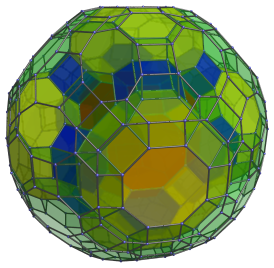For clarity, cells lying on the far side of the polytope have been omitted.

## Structure

We will explore the structure of the omnitruncated 24-cell by means of its parallel projection centered on a great rhombicuboctahedron.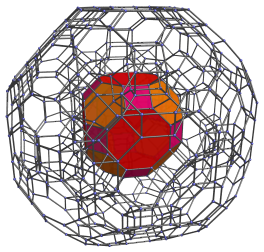The above image shows the nearest great rhombicuboctahedron to the 4D viewpoint. The hexagonal faces of this cell are joined to 8 hexagonal prisms, as shown in the next image: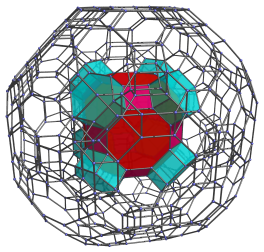The square faces of the nearest cell are joined to 12 other hexagonal prisms. The next image includes these as well: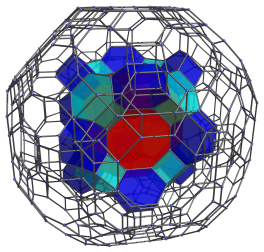The remaining octagonal faces of the nearest cell are joined to 6 other great rhombicuboctahedra, in a skewed orientation. These are shown below: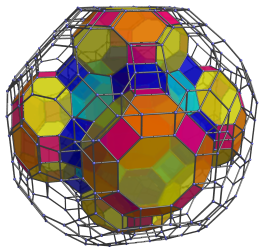Straddling these cells are 12 more hexagonal prisms, lying on top of the blue hexagonal prisms. These are shown next: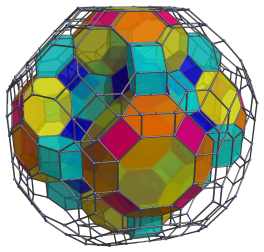In addition, the hexagonal faces of these great rhombicuboctahedra are joined to 24 more hexagonal prisms. We show them below: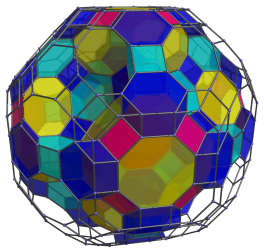Next, the square faces of these great rhombicuboctahedra are joined to yet another 24 hexagonal prisms. These are added below: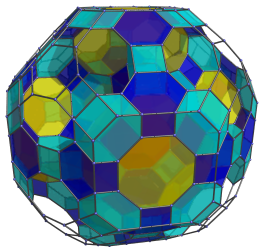Finally, the octagonal faces of the great rhombicuboctahedra, and the hexagonal and square faces of the hexagonal prisms we have seen so far, form 8 gaps into which 8 more great rhombicuboctahedral cells may be fitted. The following image shows these cells fitted in.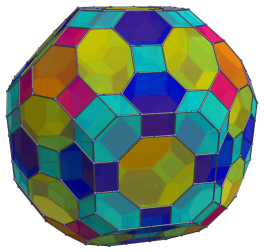This makes a total of 1+6+8 = 15 great rhombicuboctahedral cells, and 8+12+12+24+24 = 80 hexagonal prisms. These are the cells that lie on the “northern hemisphere” of the omnitruncated 24-cell.

Now we proceed to the “equator” of the omnitruncated 24-cell, where there are another 18 great rhombicuboctahedral cells. The next image shows 12 of these cells: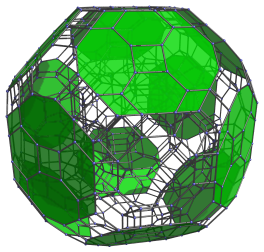The following image shows the other 6 great rhombicuboctahedral cells: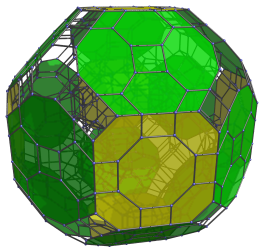There are 32 hexagonal prisms filling the remaining gaps. Eight of these are shown in the next image: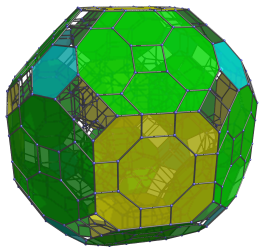Finally, the remaining hexagonal prisms are shown in the following image: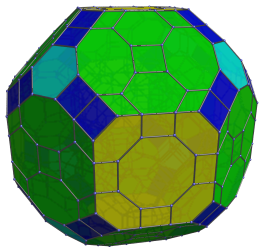These are all the cells that lie on the equator of the omnitruncated 24-cell. There are 50 of them in total.

On the far side of the omnitruncated 24-cell are the “southern hemisphere” cells, which are laid out in a structure that exactly mirrors the northern hemisphere cells. Therefore, there are 15 + 80 + 50 + 80 + 15 = 240 cells in total, with 48 great rhombicuboctahedra and 192 hexagonal prisms.

## Coordinates

The coordinates of the vertices of the omnitruncated 24-cell are all permutations of coordinates and changes of sign of the following base points:

• (1, 1+√2, 1+2√2, 5+3√2)
• (1, 3+√2, 3+2√2, 3+3√2)
• (2, 2+√2, 2+2√2, 4+3√2)

Last updated 17 Jun 2019.